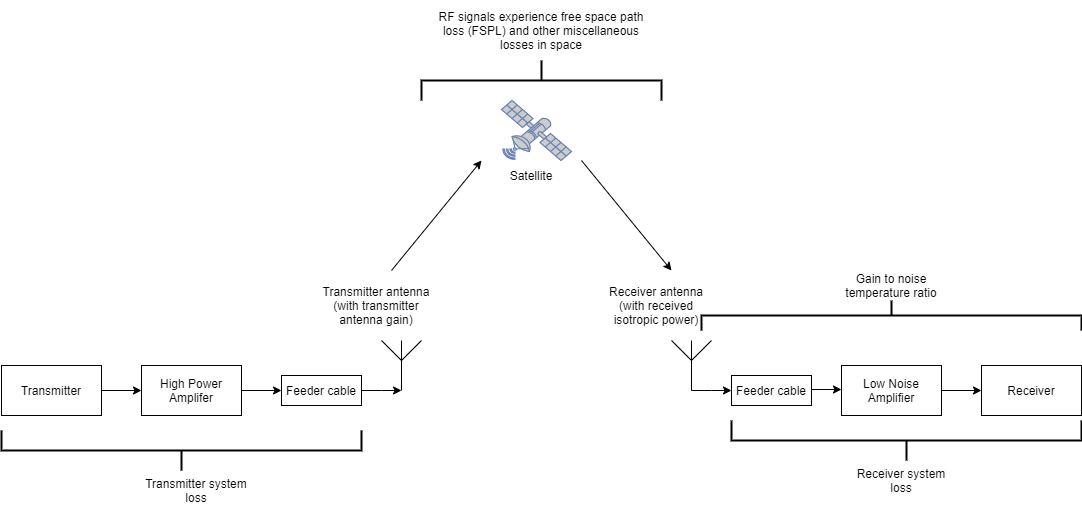# satelliteCNR

Carrier-to-noise ratio for configured satellite link budget parameters

Since R2022b

## Syntax

``[cn,info] = satelliteCNR(cfg)``

## Description

example

````[cn,info] = satelliteCNR(cfg)` computes the carrier-to-noise ratio (CNR) `cn` for the satellite link budget parameters specified in the configuration `cfg`. The function also returns the intermediate results in the CNR calculation `info` based on the link budget parameters specified in `cfg`.```

## Examples

collapse all

Create a default CNR configuration object, and then set its properties.

```cfg = satelliteCNRConfig; cfg.TransmitterPower = 17; % in dBW cfg.TransmitterSystemLoss = 9; % in dB cfg.TransmitterAntennaGain = 38; % in dBi cfg.Distance = 40215; % in km cfg.Frequency = 11; % in GHz % Here, miscellaneous losses include polarization loss, interference % loss, and antenna mispointing loss, respectively. polLoss = 3.0103; intLoss = 2; antLoss = 1; cfg.MiscellaneousLoss = polLoss + intLoss + antLoss; % in dB cfg.GainToNoiseTemperatureRatio = 25; % in dB/K cfg.ReceiverSystemLoss = 2; % in dB cfg.BitRate = 10; % in Mbps```

Display the CNR configuration object properties.

`disp(cfg)`
``` satelliteCNRConfig with properties: TransmitterPower: 17 TransmitterSystemLoss: 9 TransmitterAntennaGain: 38 Distance: 40215 Frequency: 11 MiscellaneousLoss: 6.0103 GainToNoiseTemperatureRatio: 25 ReceiverSystemLoss: 2 BitRate: 10 SymbolRate: 10 Bandwidth: 6 ```

Calculate the CNR.

`[cn,info] = satelliteCNR(cfg)`
```cn = 18.4440 ```
```info = struct with fields: TransmitterEIRP: 46 FSPL: 205.3634 ReceivedIsotropicPower: -165.3737 CarrierToNoiseDensityRatio: 86.2255 ReceivedEbNo: 16.2255 ReceivedEsNo: 16.2255 ```

Compute the link margin. Assume a required energy per bit to noise power density ratio (Eb/No) of 10 dB and an implementation loss of 2 dB in the receiver.

```reqEbNo = 10; implLoss = 2; margin = info.ReceivedEbNo - reqEbNo - implLoss```
```margin = 4.2255 ```

## Input Arguments

collapse all

CNR configuration object, specified as a `satelliteCNRConfig` object.

## Output Arguments

collapse all

Carrier-to-noise ratio in dB, returned as a scalar. For more information, see Algorithms.

Data Types: `double`

Intermediate results in the CNR calculation, based on the link budget parameters specified in the configuration object `cfg` returned as a structure with these fields.

Structure FieldDescription
`TransmitterEIRP`

Effective isotropic radiated power (EIRP) of the transmitter antenna, returned as a scalar. Value is in dBW.

`FSPL`

Free space path loss (FSPL) from the transmitter to the receiver antenna, returned as a scalar. Value is in dB.

`ReceivedIsotropicPower`

Received isotropic power at the receiver antenna, returned as a scalar. Value is in dBW.

`CarrierToNoiseDensityRatio`

Carrier-to-noise power density ratio (C/No), returned as a scalar. Value is in dB-Hz.

`ReceivedEbNo`

Received energy per bit to noise power density ratio (Eb/No), returned as a scalar. Value is in dB.

`ReceivedEsNo`

Received energy per symbol to noise power density ratio (Es/No), returned as a scalar. Value is in dB.

All fields in the structure are of data type `double`.

Data Types: `struct`

## Algorithms

The CNR for a satellite communications system measures the strength of the carrier signal at a receiver relative to the strength of the noise at that receiver. This diagram shows the various signal losses and gains involved in satellite communications.To compute the CNR `cn` for a system, the `satelliteCNR` function performs various intermediate calculations, using the properties of the `satelliteCNRConfig` configuration object, and outputs them to a structure, `info`. The function uses these formulas for the intermediate calculations.

`info` Structure FieldFormula
`TransmitterEIRP`

`TransmitterPower``TransmitterSystemLoss` + `TransmitterAntennaGain`

`FSPL`

20*log10(4*π*`Distance`*`Frequency``physconstant`(`"LightSpeed"`))

`ReceivedIsotropicPower`

`TransmitterEIRP` - `FSPL` - `MiscellaneousLoss`

`CarrierToNoiseDensityRatio`

`ReceivedIsotropicPower` + `GainToNoiseTemperatureRatio` - 10*log10(`physconstant`(`"Boltzmann"`)) - `ReceiverSystemLoss`

`ReceivedEbNo`

`CarrierToNoiseDensityRatio` - 10*log10(`BitRate`) - 60

`ReceivedEsNo`

`CarrierToNoiseDensityRatio` - 10*log10(`SymbolRate`) - 60

The function then computes the carrier-to-noise ratio using this formula:

```cn = CarrierToNoiseDensityRatio - 10*log10(Bandwidth) - 60```

## Version History

Introduced in R2022b